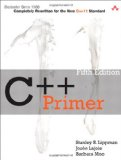# C++11 multithreading tutorial - part 2

## Posted on February 27, 2012 by Paul

The code for this tutorial is on GitHub: https://github.com/sol-prog/threads.

In my last tutorial about using threads in C++11 we’ve seen that the new C++11 threads syntax is remarkably clean compared with the POSIX pthreads syntax. Using a few simple concepts we were able to build a fairly complex image processing example avoiding the subject of thread synchronization. In the second part of this introduction to multithreading programming in C++11 we are going to see how we can synchronize a group of threads running in parallel.

We’ll start with a quick remainder of how we can create a group of threads in C++11. In the last tutorial we’ve seen that we can store a group of threads in a classical C-type array, it is entirely possible to store our threads in a std::vector which is more in the spirit of C++11 and avoids the pitfalls of dynamical memory allocation with new and delete:

Compiling the above program on Mac OSX Lion with clang++ or with gcc-4.7 (gcc-4.7 was compiled from source):

On a modern Linux system with gcc-4.7.x and up we can compile the code with:

Some real life problems are embarrassingly parallel in their nature and can be well managed with the simple syntax presented in the first part of this tutorial. Adding two arrays, multiplying an array with a scalar, generating the Mandelbroot set are classical examples of embarrassingly parallel problems.

Other problems by their nature require some level of synchronization between threads. Take for example the dot product of two vectors: take two vectors of equal lengths multiply them element by element and add the result of each multiplication in a scalar variable. A naive parallelization of this problem is presented in the next code snippet:

The result of the above code should obviously be 200000, however, running the code a few times gives slightly different results:

What has happened ??? Look carefully at line 9 of the C++ code, you can see that the variable result sums the result of v1[i] and v2[i]. Line 9 is a typical example of a race condition, this code runs in two parallel asynchronous threads and the variable result is changed by whichever thread access it first.

We can avoid this problem by specifying that this variable should be accessed synchronously by our threads, we can use for this a mutex which is a special purpose variable that acts like a barrier, synchronizing the access to the code that modifies the result variable:

Line 6 creates a global mutex variable barrier, line 15 forces the threads to finalize the for loop and access synchronously result. Notice that this time we use a new variable partial_sum declared locally for each thread. The rest of the code is unchanged.

For this particular case we can actually find a simpler and more elegant solution, we can use an atomic type which is a special kind of variable that allows safe concurrent reading/writing, basically the synchronization is done under the hood. As a side note on an atomic type we can apply only atomic operations which are defined in the atomic header:

The atomic types and atomic operations are not available in the current Apple’s clang++, however you can use atomic types if you are wiling to compile the last clang++ from sources, or you can use the last gcc-4.7 also compiled from sources.

If you are interested in learning more about the new C++11 syntax I would recommend reading Professional C++ by M. Gregoire, N. A. Solter, S. J. Kleper:or, if you are a C++ beginner you could read C++ Primer (5th Edition) by S. B. Lippman, J. Lajoie, B. E. Moo.